Step-by-Step Guide to Estimating Errors and Confidence LevelsOverview

Synopsis:

This thread uses the "native" Sherpa interface to estimate errors or confidence levels for parameters in a fit (to data of any dimensionality).

Last Update: 22 Dec 2020 - Updated for CIAO 4.13: use new plot style for PHA data

Getting Started

Finding the best fit

First, we check the current Sherpa settings. By default, Sherpa uses the Levenberg-Marquardt method to optimize the fit of a model to data with χ2 statistics, using the Gehrels variance function. In this example, we choose to fit with the default optimization method, and change the fit statistic from default to the Chi2Xspecvar statistic.Tip

The available optimization methods and statistics may be listed with list_methods and list_stats, and can be changed with set_method and set_stat. Detailed information on each option may be found in the ahelp documentation, as well as on the Sherpa Optimization Methods and Statistics pages.

sherpa> clean()
sherpa> show_method()
Optimization Method: LevMar
name     = levmar
ftol     = 1.1920928955078125e-07
xtol     = 1.1920928955078125e-07
gtol     = 1.1920928955078125e-07
maxfev   = None
epsfcn   = 1.1920928955078125e-07
factor   = 100.0
numcores = 1
verbose  = 0

sherpa> set_stat("chi2datavar")
sherpa> show_stat()
Statistic: Chi2DataVar
Chi Squared with data variance.

The variance in each bin is estimated from the data value in that
bin.

If the number of counts in each bin is large, then the shape of
the Poisson distribution from which the counts are sampled tends
asymptotically towards that of a Gaussian distribution, with
variance

sigma(i)^2 = N(i,S) + [A(S)/A(B)]^2 N(i,B)

where N is the number of on-source (and off-source) bins included
in the fit. The background term appears only if an estimate of the
background has been subtracted from the data.

A(B) is the off-source "area", which could be
the size of the region from which the background is extracted, or
the length of a background time segment, or a product of the two,
etc.; and A(S) is the on-source "area". These terms may be defined
for a particular type of data: for example, PHA data sets A(B) to
BACKSCAL * EXPOSURE from the background data set and A(S) to
BACKSCAL * EXPOSURE from the source data set.

--------
Chi2Gehrels, Chi2ModVar, Chi2XspecVar

statistical errors were found in file 'source_grouped_pi.fits'
but not used; to use them, re-read with use_errors=True

sherpa> notice(0.5, 8.0)
sherpa> plot_data()

The data we will be fitting is read into the session with the load_data command, which automatically loads the instrument response associated with a source data set when the ARF and RMF filenames are recorded in the header of the source data file, as shown above; this also applies to background data associated with the source (not considered in this example). The plot in Figure 1 results from the plot_data command above, showing the source data that is to be fit, between 0.5-8.0 keV.

Figure 1: Source spectrum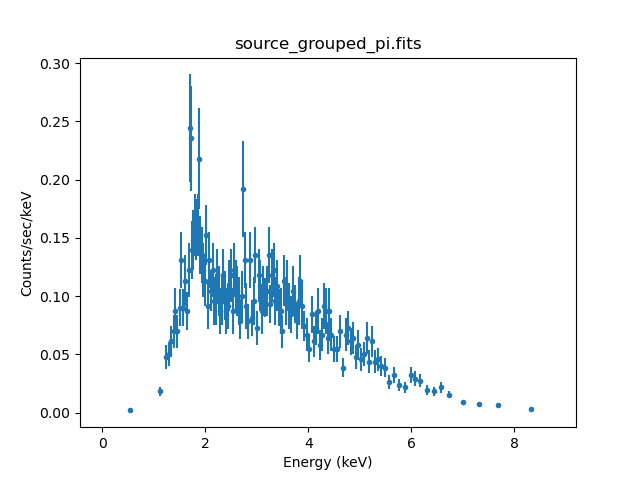Figure 1: Source spectrum

The source model is set to an absorbed power-law with the set_source command, and fit with the fit command, producing the results shown below.

sherpa> set_source(xsphabs.abs1 * powlaw1d.p1)
sherpa> fit()
Dataset               = 1
Method                = levmar
Statistic             = chi2datavar
Initial fit statistic = 6.65323e+09
Final fit statistic   = 123.621 at function evaluation 29
Data points           = 133
Degrees of freedom    = 130
Probability [Q-value] = 0.640847
Reduced statistic     = 0.950933
Change in statistic   = 6.65323e+09
abs1.nH        2.25588      +/- 0.117471
pl.gamma       1.47469      +/- 0.081887
pl.ampl        0.00185338   +/- 0.000224613

The fit and δχ residuals (also refered to as σ residuals, which is not the same σ as standard deviation) may be plotted with the plot or plot_fit_delchi command. Here we use Matplotlib commands to adjust the axes after the plot has been created, resulting in Figure 2:

sherpa> plot_fit_delchi(xlog=True, ylog=True)
sherpa> plt.xlim(0.3, 10)
(0.3, 10)

Figure 2: Best fit model with residuals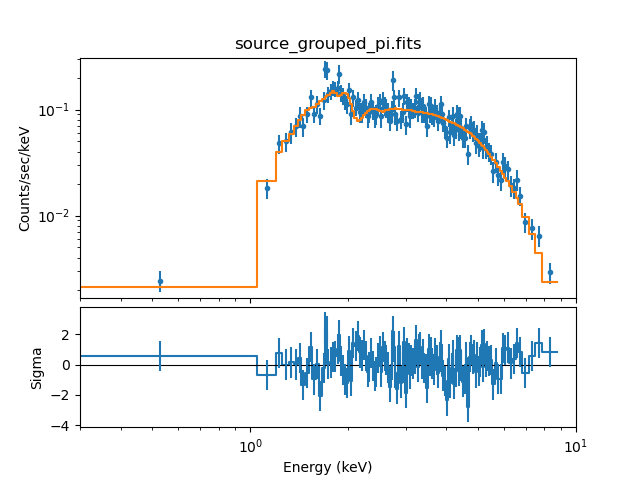Figure 2: Best fit model with residuals

New in CIAO 4.13 is the fact that residual-style plots, such as the delchi plot, now always use a linear scale for the Y axis, and so there is no-longer a need to call

plt.yscale('linear')

New in CIAO 4.12 is the ability to over-ride plot preferences in a plot call. This is used here to ensure all axes are drawn with a logarithmic scale (other than the Y axis for the residual plot!). The one adjustment is to the X axis (which affects both plots as they are "linked" together); the X axis bin goes down to a very-small value (10-3), which ends up dominating the axis due to the logarithmic scale, so a more-appropriate range is chosen with the plt.xlim call.

The show_fit and get_fit_results commands are also available for checking the quality of the fit:

sherpa> print(get_fit_results())
datasets       = (1,)
itermethodname = none
methodname     = levmar
statname       = chi2datavar
succeeded      = True
parnames       = ('abs1.nH', 'pl.gamma', 'pl.ampl')
parvals        = (2.2558840821480937, 1.4746943632186176, 0.0018533814206418062)
statval        = 123.62123838387117
istatval       = 6653225321.935967
dstatval       = 6653225198.314729
numpoints      = 133
dof            = 130
qval           = 0.6408472990001428
rstat          = 0.9509326029528552
message        = successful termination
nfev           = 29

Confidence limits for individual parameters

After finding the best-fit model parameter values, we calculate the confidence limits (parameter bounds) for these parameters using either the confidence, projection or covariance methods. The confidence limits are defined by a required confidence level in our analysis, typically the 68.3% or 90% level, corresponding to 1σ and 1.6σ for the normal distribution. The table below displays the relationship between the standard deviation, σ, and confidence level for one significant parameter. The relationship between the confidence level, Δχ2, and the general log-likelihood, $$\Delta \log{\mathcal{L}}=\Delta\chi^{2}/2$$.

Confidence intervals for a normal distribution

Confidence $$\sigma$$ $$\Delta \chi^{2}$$ $$\Delta \log{\mathcal{L}}$$
68.3% 1.0 1.00 0.50
90.0% 1.6 2.71 1.36
95.5% 2.0 4.00 2.00
99.0% 2.6 6.63 3.32
99.7% 3.0 9.00 4.50

We will use the confidence method to estimate 1σ errors on the gamma parameter of the power-law model component:

sherpa> print(get_conf())
name         = confidence
sigma        = 1
eps          = 0.01
maxiters     = 200
soft_limits  = False
remin        = 0.01
fast         = False
parallel     = True
numcores     = 4
maxfits      = 5
max_rstat    = 3
tol          = 0.2
verbose      = False
openinterval = False

sherpa> conf(p1.gamma)
pl.gamma lower bound:   -0.0830705
pl.gamma upper bound:   0.0849456
Dataset               = 1
Confidence Method     = confidence
Iterative Fit Method  = None
Fitting Method        = levmar
Statistic             = chi2datavar
confidence 1-sigma (68.2689%) bounds:
Param            Best-Fit  Lower Bound  Upper Bound
-----            --------  -----------  -----------
pl.gamma          1.47469   -0.0830705    0.0849456

To access the 90% confidence limits on a parameter, the sigma field of the conf_opt variable should be changed to 1.6.

sherpa> set_conf_opt("sigma", 1.6)
sherpa> conf(p1.gamma, abs1.nH)
abs1.nH lower bound:    -0.183538
abs1.nH upper bound:    0.197266
pl.gamma lower bound:   -0.13186
pl.gamma upper bound:   0.136806
Dataset               = 1
Confidence Method     = confidence
Iterative Fit Method  = None
Fitting Method        = levmar
Statistic             = chi2datavar
confidence 1.6-sigma (89.0401%) bounds:
Param            Best-Fit  Lower Bound  Upper Bound
-----            --------  -----------  -----------
pl.gamma          1.47469     -0.13186     0.136806
abs1.nH           2.25588    -0.183538     0.197266

We have also used conf to calculate the uncertainty on the optimized hydrogen column density parameter of the absorption model, abs1.nH.

To estimate errors on all the thawed parameters, conf should be called with no parameter names. Since sigma is still set to 1.6 for the confidence method, the following calculates the 90% confidence limits for all the thawed parameters:

sherpa> conf()
abs1.nH lower bound:    -0.183538
abs1.nH upper bound:    0.197266
pl.ampl lower bound:    -0.000329436
pl.gamma lower bound:   -0.13186
pl.gamma upper bound:   0.136806
pl.ampl upper bound:    0.000413355
Dataset               = 1
Confidence Method     = confidence
Iterative Fit Method  = None
Fitting Method        = levmar
Statistic             = chi2datavar
confidence 1.6-sigma (89.0401%) bounds:
Param            Best-Fit  Lower Bound  Upper Bound
-----            --------  -----------  -----------
abs1.nH           2.25588    -0.183538     0.197266
pl.gamma          1.47469     -0.13186     0.136806
pl.ampl        0.00185338 -0.000329436  0.000413355

The covariance command behaves similarly to conf, although the fields in the state object are different. While it is quicker than the confidence method, it is less accurate; i.e., it is always symmetric because it uses the diagonal elements of the covariance matrix and ignores correlations between the parameters.

Note that the computationally intensive confidence function has been parallelized in Sherpa, to make use of multi-core systems (i.e., laptops or desktops with 2 or more cores).

Common WARNING messages returned by confidence methods

WARNING: hard minimum hit for parameter <parameter name>

When the confidence, projection, and covariance methods are used to estimate confidence intervals for thawed model parameters after a fit, sometimes a hard upper or lower limit will be reached for one or more parameter. This produces the message "WARNING: hard minimum hit for parameter <parameter name>", along with a row of dashes in the appropriate place in the function output.Note

The covariance method can also return a null value for an upper/lower limit when the parameter space at the minimum is non-quadratic for a given parameter. The covariance matrix calculations assume that the parameters follow the normal distribution. If the parameter space is non-smooth, then the covariance calculations fail and Sherpa returns "-----".

Example confidence output:

sherpa> conf()
...

WARNING: hard minimum hit for parameter bpow1.gamma2
WARNING: hard maximum hit for parameter bpow1.gamma2
WARNING: hard minimum hit for parameter bpow1.eb
WARNING: hard maximum hit for parameter bpow1.eb
...

Dataset               = 1
Confidence Method     = confidence
Iterative Fit Method  = None
Statistic             = cstat
confidence 1-sigma (68.2689%) bounds:
Param            Best-Fit  Lower Bound  Upper Bound
-----            --------  -----------  -----------
bpow1.gamma1       1.54147   -0.0292891   0.0292709
bpow1.gamma2       8.10056        -----       -----
bpow1.eb           9.49083       -----        -----
bpow1.ampl         0.022806 -0.000378395  0.000383854

This occurs when the parameter bound found by one of the confidence methods lies outside the hard limit boundary for a model parameter—this could result from an issue with the signal-to-noise of the data, the applicability of the model to the data, systematic errors in the data, among others things.

A parameter hard limit represents either a hard physical limit (e.g., temperature is not allowed to go below zero), a mathematical limit (e.g., prevent a number from going to zero or below, when the log of that number will be taken), or the limit of what a float or double can hold (the fit should not be driven above or below the maximum or minimum values a variable can hold). For this reason, model parameter hard limits should not be changed by the user.

WARNING: The confidence level lies within <interval>

Another warning message which may be returned by confidence is that a model parameter lies within the stated range:

sherpa>  conf(g15.Sigma)
...
g15.Sigma -: WARNING: The confidence level lies within (8.706380e-05,9.252185e-05)
...
Datasets              = 1, 2
Confidence Method     = confidence
Iterative Fit Method  = None
Fitting Method        = levmar
Statistic             = chi2datavar
confidence 1.64-sigma (89.8995%) bounds:
Param            Best-Fit  Lower Bound  Upper Bound
-----            --------  -----------  -----------
g15.Sigma     0.000997626 -0.000907834  0.000597058

This occurs where confidence cannot locate the root (minimum value of the fit statistic function) even though the root is bracketed within an interval (perhaps due to poor resolution of the data or a discontinuity). In such cases, when the openinterval option of confidence is set to False (default), the confidence function will not be able to find the root within the set tolerance and the function will return the average of the open interval which brackets the root. If the option openinterval is set to True, then confidence will print the minimal open interval which brackets the root (not to be confused with the lower and upper bound of the confidence interval). The most accurate thing to do is to return an open interval where the root is localized/bracketed rather than the average of the open interval (since the average of the interval is not a root within the specified tolerance).

The output from confidence may be checked by setting 'set_conf_opt('verbose',1)', and then re-running confidence for the relevant parameter(s).

sherpa> set_conf_opt('verbose',1)
sherpa> conf(g15.Sigma)
#
# f[  2.931742e+00   2.957942e-02   1.471941e+00   9.976265e-04
1.851972e-04
1.840837e+00   3.667986e-03   7.820442e-05   1.864564e+00   2.562143e-03
1.415699e-04   2.004898e+00   6.288115e-04   1.259512e-04] =
8.569929e+02
# sigma = 1.640000e+00
# target_stat = 8.596825e+02
# tol = 1.000000e-02
# smin = [-2.    0.    1.45  0.    0.    1.82  0.    0.    1.85  0.
0.    1.96
0.    0.  ]
# smax = [  9.000000e+00   1.000000e+24   1.500000e+00   1.000000e-02
1.000000e-03
1.850000e+00   1.000000e-02   1.000000e-03   1.900000e+00   1.000000e-02
1.000000e-03   2.040000e+00   1.000000e-03   1.000000e-03]
# hmin = [ -3.402823e+38   0.000000e+00   0.000000e+00   0.000000e+00
0.000000e+00
0.000000e+00   0.000000e+00   0.000000e+00   0.000000e+00   0.000000e+00
0.000000e+00   0.000000e+00   0.000000e+00   0.000000e+00]
# hmax = [  3.402823e+38   3.402823e+38   3.402823e+38   3.402823e+38
3.402823e+38
3.402823e+38   3.402823e+38   3.402823e+38   3.402823e+38   3.402823e+38
3.402823e+38   3.402823e+38   3.402823e+38   3.402823e+38]
#
# Note: for the intermediate steps, the notation:
par.name -/+: f( x ) = stat
# ==> stat is the statistic when parameter par.name is frozen at x
# while searching for the lower/upper confidence level, repectively.
#
g15.Sigma -: f( 4.812517e-04 ) = -2.565633e+00
g15.Sigma -: f( 0.000000e+00 ) = 3.505966e+01
g15.Sigma -: f( 2.406259e-04 ) = -2.565434e+00
g15.Sigma -: f( 2.113694e-04 ) = -2.565393e+00
g15.Sigma -: f( 1.653199e-04 ) = -2.565381e+00
g15.Sigma -: f( 1.343754e-04 ) = -2.564794e+00
g15.Sigma -: f( 1.079722e-04 ) = -2.565100e+00
g15.Sigma -: f( 8.706380e-05 ) = 3.778055e+01
g15.Sigma -: f( 1.068516e-04 ) = -2.563338e+00
g15.Sigma -: f( 1.022595e-04 ) = -2.562500e+00
g15.Sigma -: f( 9.961908e-05 ) = -2.563027e+00
g15.Sigma -: f( 9.721570e-05 ) = -2.564524e+00
g15.Sigma -: f( 9.532733e-05 ) = -2.562276e+00
g15.Sigma -: f( 9.377811e-05 ) = -2.562519e+00
g15.Sigma -: f( 9.252185e-05 ) = -2.563134e+00
g15.Sigma -: f( 9.149979e-05 ) = -2.563034e+00
g15.Sigma -: f( 9.066944e-05 ) = -2.563196e+00
g15.Sigma -: WARNING: The confidence level lies within (8.706380e-05,
9.252185e-05)
g15.Sigma lower bound:  -0.000907834
g15.Sigma +: f( 1.514001e-03 ) = -8.109195e-01
g15.Sigma +: f( 2.546751e-03 ) = 2.553184e+01
g15.Sigma +: f( 2.030376e-03 ) = 7.845440e+00
g15.Sigma +: f( 2.030376e-03 ) = 7.845440e+00
g15.Sigma +: f( 2.030376e-03 ) = 7.845440e+00
g15.Sigma +: f( 1.772189e-03 ) = 2.467092e+00
g15.Sigma +: f( 1.772189e-03 ) = 2.467092e+00
g15.Sigma +: f( 1.772189e-03 ) = 2.467092e+00
g15.Sigma +: f( 1.643095e-03 ) = 5.832027e-01
g15.Sigma +: f( 1.643095e-03 ) = 5.832027e-01
g15.Sigma +: f( 1.643095e-03 ) = 5.832027e-01
g15.Sigma +: f( 1.578548e-03 ) = -1.721177e-01
g15.Sigma +: f( 1.578548e-03 ) = -1.721177e-01
g15.Sigma +: f( 1.578548e-03 ) = -1.721177e-01
g15.Sigma +: f( 1.610822e-03 ) = 1.906909e-01
g15.Sigma +: f( 1.610822e-03 ) = 1.906909e-01
g15.Sigma +: f( 1.610822e-03 ) = 1.906909e-01
g15.Sigma +: f( 1.594685e-03 ) = 5.746982e-03
g15.Sigma upper bound:  0.000597058
Datasets              = 1, 2
Confidence Method     = confidence
Iterative Fit Method  = None
Fitting Method        = levmar
Statistic             = chi2datavar
confidence 1.64-sigma (89.8995%) bounds:
Param            Best-Fit  Lower Bound  Upper Bound
-----            --------  -----------  -----------
g15.Sigma     0.000997626 -0.000907834  0.000597058
method = levmar, stat = chi2datavar, in 17.921696 secs

How does the fit surface vary for a parameter (interval-projection)?

In order to visually inspect the model parameter space we can "project" the statistics into the 1-D or 2-D plane with int_proj and reg_proj, respectively. This allows for checking the shape of the parameter space around the best fit parameter values, as well as evaluate correlations between parameters.

Here we use the int_proj (interval-projecton) method to see how the fit statistic varies with the gamma parameter of the power-law component. Since we already know that the 90% errors of p1.gamma are approximately $$\pm 0.13$$, we choose to set the axis range manually:

sherpa> print(get_int_proj())
x     = None
y     = None
min   = None
max   = None
nloop = 20
delv  = None
fac   = 1
log   = False

sherpa> int_proj(p1.gamma, min=1, max=2)
sherpa> print(get_int_proj())
x     = [1.    ,1.0526,1.1053,1.1579,1.2105,1.2632,1.3158,1.3684,1.4211,1.4737,
1.5263,1.5789,1.6316,1.6842,1.7368,1.7895,1.8421,1.8947,1.9474,2.    ]
y     = [159.736 ,151.8122,144.9428,139.0959,134.2386,130.3377,127.3595,125.27  ,
124.0353,123.6214,123.9943,125.1203,126.9661,129.4985,132.685 ,136.4935,
140.8926,145.8515,151.3402,157.3294]
min   = 1
max   = 2
nloop = 20
delv  = None
fac   = 1
log   = False

The resulting plot is shown in Figure 3.

Figure 3: Plot of interval-projection results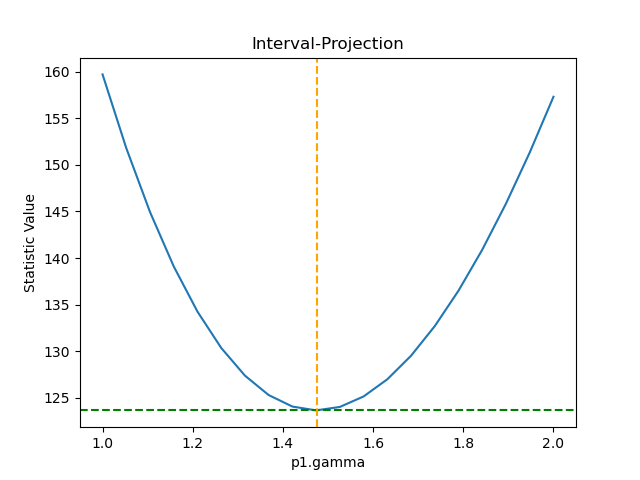Figure 3: Plot of interval-projection results

Confidence interval plot of the fit statistic versus the p1.gamma model parameter value.

The "confidence intervals" table above lists a range of common confidence levels and the corresponding change in χ2 values (i.e., the statistic value on the y-axis in this plot). The parameters displayed by print(get_int_proj()) show how the fit statistic versus single model parameter plot is calculated. The parameters min and max are the minimum and maximum grid boundary values; if set to the default values of None, the grid boundaries are calculated automatically from the covariance. nloop is the bin size, which by default is used with the min and max grid boundaries in order to determine the step size, delv (default is delv=None).

The int_unc (interval-uncertainty) command behaves similarly to int_proj , although the fields in the state object for the two methods are different.

How are two parameters correlated (region-projection)?

In this section we use the reg_proj (region-projection) method of Sherpa to see whether the p1.gamma and abs1.nh parameters are correlated.

From our earlier run we know that the 90% errors on the two parameters—when evaluated independently—are approximately 0.14 (gamma) and 0.2 (nH). However we decide to let the routine calculate plot limits automatically, and choose to display contours at the 1 and 1.6 σ level (68.3% and 90% confidence levels).

sherpa> print(get_reg_proj())
x0      = None
x1      = None
y       = None
min     = None
max     = None
nloop   = (10, 10)
fac     = 4
delv    = None
log     = (False, False)
sigma   = (1, 2, 3)
parval0 = None
parval1 = None
levels  = None

sherpa> reg_proj(p1.gamma, abs1.nH, sigma=[1, 1.6])

The resulting plot is shown in Figure 4.

Figure 4: Plot of region-projection results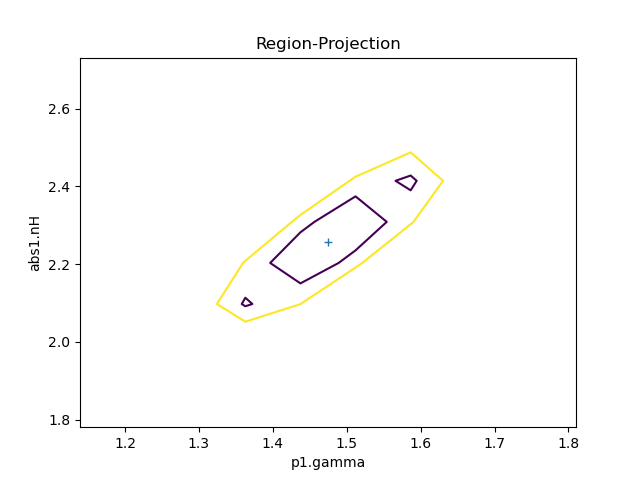Figure 4: Plot of region-projection results

This plot shows the results of reg_proj() (confidence contour of fit statistic vs. two thawed parameter values), using the default values: 10 points were used along each axis and the range was calcualted automatically. The two contours are drawn at the 68.3% and 90% confidence levels. The plot needs to be re-evaluated using more points, and with a better choice of the axes.

sherpa> print(get_reg_proj())
x0      = [1.1367,1.2118,1.2869,1.362 ,1.4371,1.5122,1.5874,1.6625,1.7376,1.8127,
1.1367,1.2118,1.2869,1.362 ,1.4371,1.5122,1.5874,1.6625,1.7376,1.8127,
1.1367,1.2118,1.2869,1.362 ,1.4371,1.5122,1.5874,1.6625,1.7376,1.8127,
1.1367,1.2118,1.2869,1.362 ,1.4371,1.5122,1.5874,1.6625,1.7376,1.8127,
1.1367,1.2118,1.2869,1.362 ,1.4371,1.5122,1.5874,1.6625,1.7376,1.8127,
1.1367,1.2118,1.2869,1.362 ,1.4371,1.5122,1.5874,1.6625,1.7376,1.8127,
1.1367,1.2118,1.2869,1.362 ,1.4371,1.5122,1.5874,1.6625,1.7376,1.8127,
1.1367,1.2118,1.2869,1.362 ,1.4371,1.5122,1.5874,1.6625,1.7376,1.8127,
1.1367,1.2118,1.2869,1.362 ,1.4371,1.5122,1.5874,1.6625,1.7376,1.8127,
1.1367,1.2118,1.2869,1.362 ,1.4371,1.5122,1.5874,1.6625,1.7376,1.8127]
x1      = [1.7772,1.7772,1.7772,1.7772,1.7772,1.7772,1.7772,1.7772,1.7772,1.7772,
1.8836,1.8836,1.8836,1.8836,1.8836,1.8836,1.8836,1.8836,1.8836,1.8836,
1.9899,1.9899,1.9899,1.9899,1.9899,1.9899,1.9899,1.9899,1.9899,1.9899,
2.0963,2.0963,2.0963,2.0963,2.0963,2.0963,2.0963,2.0963,2.0963,2.0963,
2.2027,2.2027,2.2027,2.2027,2.2027,2.2027,2.2027,2.2027,2.2027,2.2027,
2.309 ,2.309 ,2.309 ,2.309 ,2.309 ,2.309 ,2.309 ,2.309 ,2.309 ,2.309 ,
2.4154,2.4154,2.4154,2.4154,2.4154,2.4154,2.4154,2.4154,2.4154,2.4154,
2.5218,2.5218,2.5218,2.5218,2.5218,2.5218,2.5218,2.5218,2.5218,2.5218,
2.6282,2.6282,2.6282,2.6282,2.6282,2.6282,2.6282,2.6282,2.6282,2.6282,
2.7345,2.7345,2.7345,2.7345,2.7345,2.7345,2.7345,2.7345,2.7345,2.7345]
y       = [143.7825,145.3247,155.4125,174.083 ,201.2284,236.5969,279.7989,330.3201,
387.5388,450.7476,141.6868,135.3785,137.0297,146.7888,164.6786,190.5924,
224.294 ,265.4248,313.5139,367.9944,147.9872,135.0252,129.4146,131.3832,
141.0527,158.4311,183.4096,215.7637,255.1592,301.1615,160.7831,142.2249,
130.4162,125.6386,128.0855,137.8532,154.9347,179.2178,210.4865,248.4261,
178.5517,155.3186,138.257 ,127.6825,123.8383,126.8861,136.8982,153.8533,
177.6349,208.033 ,200.0785,172.9645,151.4788,135.9554,126.6704,123.8318,
127.5718,137.9409,154.9039,178.3398,224.3977,194.0822,168.8918,149.1679,
135.2055,127.2444,125.4603,129.9586,140.7695,157.8453,250.7438,217.8051,
189.5302,166.2596,148.2976,135.9034,129.2832,128.5836,133.8854,145.2004,
278.5116,243.4399,212.6123,186.3627,164.9971,148.7852,137.9532,132.6771,
133.0762,139.2095,307.2241,270.4337,237.5076,208.7693,184.521 ,165.0362,
150.5527,141.2664,137.3254,138.8248]
min     = [1.1366511  1.77720269]
max     = [1.81270991 2.73452195]
nloop   = (10, 10)
fac     = 4
delv    = None
log     = [False False]
sigma   = [1, 1.6]
parval0 = 1.4746805027612861
parval1 = 2.2558623214598748
levels  = [125.91698772 128.04310045]

The automatically-chosen limits have resulted in a poor-quality plot: there are not enough data points close to the best-fit location. The easiest way to improve on this is to change and re-run the function, increasing the number of points. We also elect to use a smaller parameter range along both axes to reduce the amount of wasted computation. In a complex case with a larger grid, it may be worthwhile to manually set the limits before running reg_proj, since it may take longer to create a plot.

sherpa> reg_proj(p1.gamma, abs1.nH, min=[1.2, 1.9], max=[1.8, 2.6], nloop=[51, 51], sigma=[1, 1.6])

The resulting plot is shown in Figure 5, which is a smooth contour plot.

Figure 5: Improved region-projection results (nloop=[51, 51])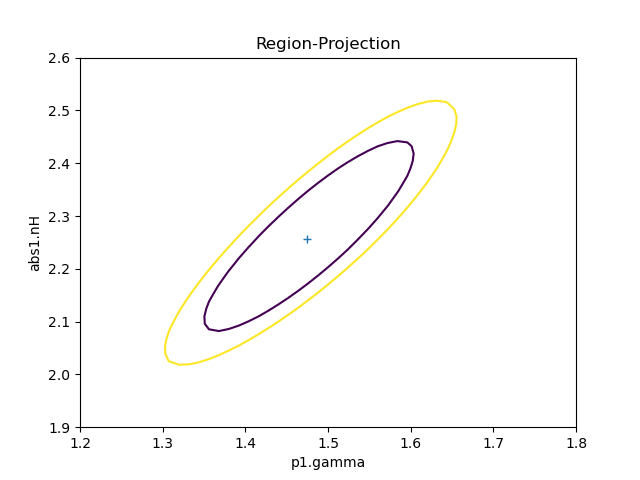Figure 5: Improved region-projection results (nloop=[51, 51])

By increasing the nloop field to 51 for each axis we have been able to create a sensible-looking contour plot.

When we utilize print(get_reg_proj()), we are given information on the most recent confidence contour plot produced by reg_proj. The min and max lists of grid boundaries are calculated automatically from the covariance when they are set to the default "None". However, they may be set manually as min=[xmin,ymin] and max=[xmax,ymax]. nloop is a list of bin sizes of the x- and y-axes, which by default are used with the min and max grid boundaries to determine the x- and y-axes step sizes, given as the list, delv. The parameter of most interest for reg_proj is sigma, which is a list of the number of σ; at which to plot contours. The levels parameter is subsequently determined after executing reg_proj, which are the confidence level z-values for each σ.Log-space for int_proj and reg_proj

In the current version of Sherpa, the log parameter should be left at its default value of False in int_proj and reg_proj, as the tools do not properly scale plots with logarithmic spacing.

The reg_unc (region-uncertainty) command behaves similarly, although the fields in the state object for the two methods are different. The two commands differ in that reg_unc fixes all other thawed parameters to their best-fit values, rather than being allowed to float to new best-fit values as in reg_proj. This makes reg_unc contours less accurate, but quicker to create.

Scripting It

The file fit.py is a Python script which performs the primary commands used above; it can be executed by typing %run -i fit.py on the Sherpa command line.

The Sherpa script command may be used to save everything typed on the command line in a Sherpa session:

sherpa> script(filename="sherpa.log", clobber=False)

(Note that restoring a Sherpa session from such a file could be problematic since it may include syntax errors, unwanted fitting trials, et cetera.)

History

 14 Jan 2005 reviewed for CIAO 3.2: no changes 21 Dec 2005 reviewed for CIAO 3.3: no changes 01 Dec 2006 reviewed for CIAO 3.4: no changes 02 Dec 2008 reviewed for CIAO 4.1: updated syntax for CIAO4.1 29 Apr 2009 new script command is available with CIAO 4.1.2 21 Jan 2010 updated for CIAO 4.2: the conf command is available 13 Jul 2010 updated for CIAO 4.2 Sherpa v2: removal of S-Lang version of thread. 15 Jul 2010 updated to include information about warning messages returned by the confidence method. 03 Sep 2010 figures moved inline with text 30 Jan 2012 reviewed for CIAO 4.4 (no changes) 13 Dec 2012 reviewed for CIAO 4.5 (no changes) 11 Dec 2013 reviewed for CIAO 4.6: updated formatting, content unchanged 18 Mar 2015 reviewed for CIAO 4.7; updated plots and fixed typos, no content change. 10 Dec 2015 reviewed for CIAO 4.8; no content change. 03 Nov 2016 reviewed for CIAO 4.9; updated outputs and fixed typos. 01 Jun 2018 reviewed for CIAO 4.10; no content change. 01 Jun 2018 reviewed for CIAO 4.11; updated screen output and added information about the confidence interval table. 12 Dec 2019 Updated for CIAO 4.12: switched from ChIPS to Matplotlib for plotting. 22 Dec 2020 Updated for CIAO 4.13: use new plot style for PHA data